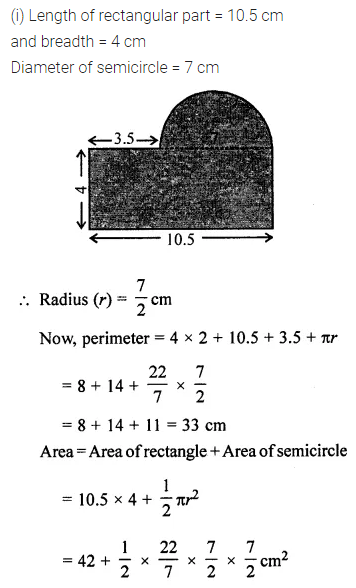# ML Aggarwal Class 7 Solutions for ICSE Maths Chapter 16 Perimeter and Area Ex 16.3

## ML Aggarwal Class 7 Solutions for ICSE Maths Chapter 16 Perimeter and Area Ex 16.3

Take π = $$\frac { 22 }{ 7 }$$, unless stated otherwise.
Question 1.
Find the circumference of the circles with the following radius:
(i) 7 cm
(ii) 21 cm
(iii) 28 mm
(iv) 3.5 cm
Solution: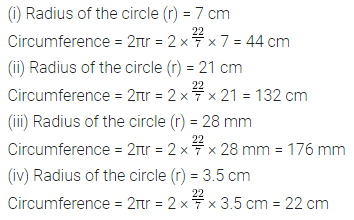Question 2.
Find the area of the circles, given that:
(ii) diameter = 49 m
(iii) diameter = 9.8 m
Solution: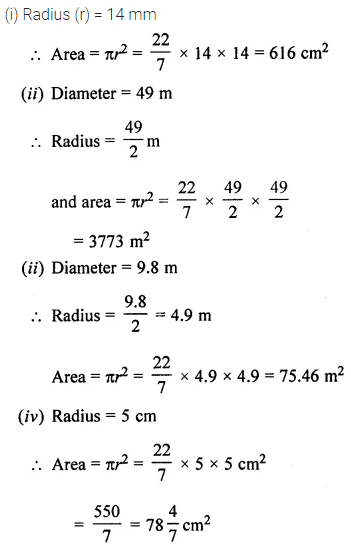Question 3.
Find the circumference and area of a circle of radius 20 cm. (use π = 3.14)
Solution: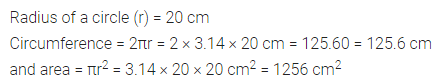Question 4.
The minute hand of a tower clock is 1.4 m long. How far does the tip of the hand move in 1 hour?
Solution:Question 5.
A gardener wants to fence a circular garden of diameter 21 m. Find the length of the rope he needs to purchase if he makes 2 rounds of the fence. Also find the cost of the rope, if it costs ₹ 4 per metre.
Solution:Question 6.
If the circumference of a circle exceeds its diameter by 30 cm, find the radius of the circle.
Solution:Question 7.
Find the length of the diameter of a circle whose circumference is 44 cm.
Solution: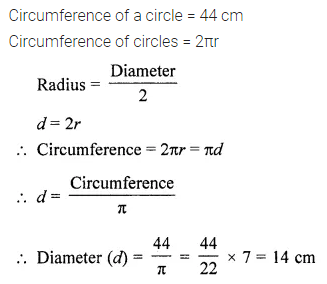Question 8.
The circumference of a circle is 31.4 cm. Find the radius and the area of the circle. (Take π = 3.14)
Solution:Question 9.
Find the radius and the circumference of a circle whose area is 144π cm2.
Solution: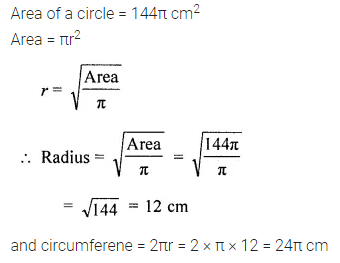Question 10.
How many times will the wheel of a car rotate in a journey of 88 km, given that the diameter of the wheel is 56 cm?
Solution:Question 11.
From square cardboard of side 21 cm, a circle of the maximum area is cut out. Find the area of the cardboard left.
Solution: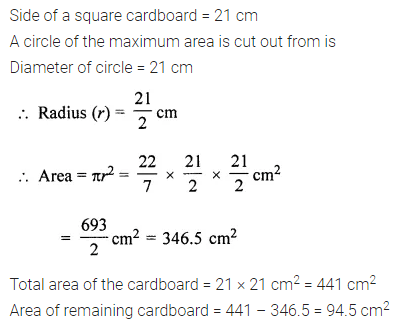Question 12.
A piece of wire is bent in the shape of an equilateral triangle of side 4.4 cm. If this wire is rebent to form of a circle, find the radius and the area of the circle.
Solution: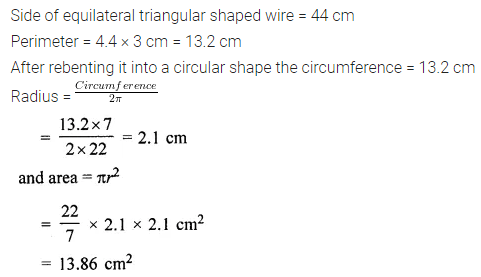Question 13.
A wire is in the form of a square of side 27.5 cm. It is straightened and bent into the shape of a circle. Find the area of the circle.
Solution:Question 14.
A wire is in the form of a rectangle 18.7 cm long and 14.3 cm wide. If this wire is reshaped and bent in the form of the circle, find the radius and the area of the circle so formed.
Solution:Question 15.
The diameter of a circular park is 84 m. On its outside, there a 3.5 m wide road. Find the cost of constructing the road at ₹ 240 per m2.
Solution:Question 16.
A circular pond is surrounded by a 2 m wide circular path. If the outer circumference of the circular path is 44 m, find the inner circumference of the circular path. Also, find the area of the path.
Solution:Question 17.
In the given figure, the area enclosed between the concentric circles is 770 cm2. If the radius of the outer circle is 21 cm, calculate the radius of the inner circle.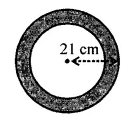Solution:Question 18.
From a circular card sheet of radius 14 cm, two circles of radius 3.5 cm and a rectangle of length 3 cm and breadth 1 cm are removed (as shown in the given figure). Find the area of the remaining sheet.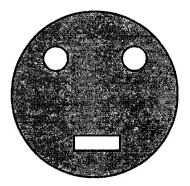Solution: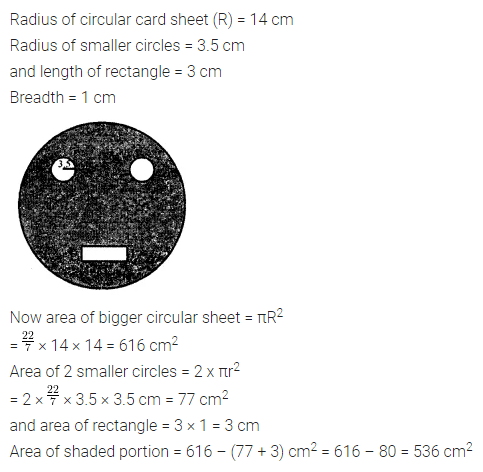Question 19.
Calculate the length of the boundary and the area of the shaded region in the following diagrams. All measurements are in centimetres.
(i) The unshaded part is a semicircle.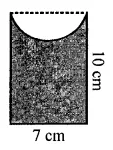(ii) Four semicircles on a square.Solution: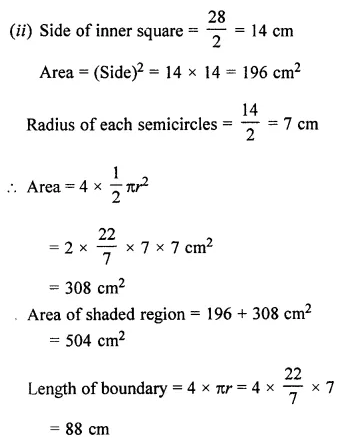Question 20.
Find the perimeter and the area of the shaded region in the following figures. All measurements are in centimetres.Solution: